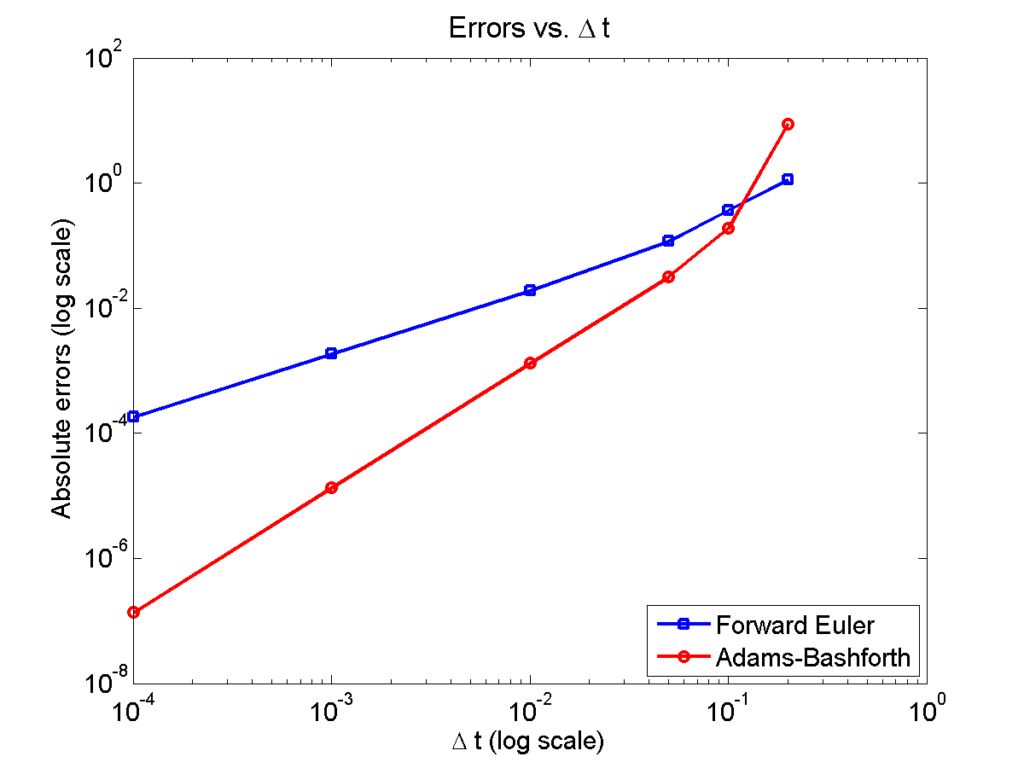## Some quick math to calculate numerical convergence ratesI find myself needing to re-derive this often enough that it’s worth jotting down for current and future students. :-)

### Introduction

A very common task in our field is to assess the convergence rate of a numerical algorithm: if I shrink $$\Delta t$$ (or $$\Delta x$$), how quickly does my error shrink? And in fact, does my error shrink? Assuming you have a method to compute the error for a simulation (say, a simple test case where you know the exact solution), you want a fit an expression like this:

$\mathrm{Error}(\Delta t) = C \Delta t^n ,$ where $$C$$ is a constant, and $$n$$ is the order of convergence. Usually, if $$n$$ isn’t at least 1, it’s bad.

So, suppose you are testing an algorithm, and you have the error $$E_1$$ for $$\Delta t_1$$ and $$E_2$$ for $$\Delta t_2$$. Then one way to go about this calculation is to try to cancel out $$C$$:

\begin{eqnarray} \frac{ E_1}{E_2} = \frac{C \Delta t_1^n }{C \Delta t_2^n } = \left( \frac{ \Delta t_1 }{\Delta t_2} \right)^n & \Longrightarrow & n = \frac{ \log\left( E_1 / E_2 \right) }{ \log\left( \Delta t_1 / \Delta t_2 \right) } \end{eqnarray}

Another way to look at this problem is to rewrite the error equation in log-log space:

\begin{eqnarray} E = C \Delta t^N & \Longrightarrow & \log{E} = \log{C} + n \log{ \Delta t} \end{eqnarray}
so $$n$$ is the slope of the equation in log space. If you only have two points, then,
$n = \frac{ \log{E_1} – \log{E_2} }{ \log{\Delta t_1} – \log{\Delta t_1} } = \frac{ \log\left( E_1 / E_2 \right) }{ \log\left( \Delta t_1 / \Delta t_2 \right) },$ and so we end up with the exact same convergence rate as before.

However, if you have calculated the error $$E_i$$ for a whole bunch of values $$\Delta t_i$$, then you can extend this idea to get a better sense of the overall convergence rate for all your values of $$\Delta t$$, rather than just two values. Just find the linear least squares fit to the points $$\left\{ ( \log\Delta t_i, \log E_i ) \right\}$$. If there are just two points, it’s the same as above. If there are many, it’s a better representation of overall convergence.

### Trying it out

Let’s demonstrate this on a simple test problem:

$\frac{du}{dt} = -10 u, \hspace{.5in} u(0) = 1.$

We’ll simulate using (1st-order) forward Euler and (2nd-order) Adams-Bashforth:

dt_vals = [.2 .1 .05 .01 .001 .0001];

min_t = 0;
max_t = 1;

lambda = 10;
initial = 1;

errors_forward_euler = [];

for j=1:length( dt_vals )
dt = dt_vals(j);
T = min_t:dt:max_t;

% allocate memory

solution_forward_euler = zeros(1,length(T));
solution_forward_euler(1) = initial;

% exact solution

solution_exact = initial * exp( -lambda * T );

% forward euler

for i=2:length(T)
solution_forward_euler(i) = solution_forward_euler(i-1)...
- dt*lambda*solution_forward_euler(i-1);
end

% adams-bashforth -- use high-res Euler to jump-start

dt_fine = dt * 0.1;
t = min_t + dt_fine;
temp = initial ;
for i=1:10
temp = temp - dt_fine*lambda*temp;
end

for i=3:length(T)
end

%     Uncomments if you want to see plots.
%     figure(1)
%     clf;
%     plot( T, solution_exact, 'r*' , T , solution_forward_euler,...
%         'b-o', T , solution_adams_bashforth , 'k-s'  );
%     pause ;

errors_forward_euler(j) = ...
max(abs( solution_exact - solution_forward_euler ) );
max(abs( solution_exact - solution_adams_bashforth ) );

end


Here is a plot of the errors:

figure(2)
loglog( dt_vals, errors_forward_euler, 'b-s' ,...
'linewidth', 2 );
legend( 'Forward Euler', 'Adams-Bashforth' , 4 );
xlabel( '\Delta t (log scale)' , 'fontsize', 14 );
ylabel( 'Absolute errors (log scale)', 'fontsize', 14 );
title( 'Errors vs. \Delta t', 'fontsize' , 16 );
set( gca, 'fontsize' , 14 );Note that calculating the convergence rate based on the first two errors, and first and last errors, is not terribly
representative, compared with using all the errors:

% Convergence rate based on the first two errors
polyfit( log(dt_vals(1:2)) , log(errors_forward_euler(1:2)) , 1 )
polyfit( log(dt_vals(1:2)) , log(errors_adams_bashforth(1:2)) , 1 )

% Convergence rate based on the first and last errors
m = length(dt_vals);
polyfit( log( [dt_vals(1),dt_vals(m)] ) ,...
log( [errors_forward_euler(1),errors_forward_euler(m)]) , 1 )
polyfit( log( [dt_vals(1),dt_vals(m)] ) ,...

% Convergence rate based on all the errors
polyfit( log(dt_vals) , log(errors_forward_euler) , 1 )
polyfit( log(dt_vals) , log(errors_adams_bashforth) , 1 )

% Convergence rate based on all the errors but the outlier
polyfit( log(dt_vals(2:m)) , log(errors_forward_euler(2:m)) , 1 )
polyfit( log(dt_vals(2:m)) , log(errors_adams_bashforth(2:m)) , 1 )


Using the first two errors gives a convergence rate over 5 for Adams-Bashforth, and around 1.6 for forward Euler. Using first and last is better, but still over-estimates the convergence rates (1.15 and 2.37, FE and AB, respectively). Linear least squares is closer to reality: 1.12 for FE, 2.21 for AB. And lastly, linear least squares but excluding the outliers, we get 1.08 for forward Euler, and 2.03 for Adams-Bashforth. (As expected!)

### Which convergence rates are “right”?

So, which values do you report as your convergence rates? Ideally, use all the errors to avoid bias and/or cherry-picking. It’s the most honest and straightforward way to present the work. However, you may have a good rationale to exclude the clear outliers in this case. But then again, if you have calculated the errors for enough values of $$\Delta t$$, there’s no need to do this at all. There’s little value in (or basis for) reporting the convergence rate to three significant digits. I’d instead report these as approximately first-order convergence (forward Euler) and approximately second-order convergence (Adams-Bashforth); we get this result with either linear least squares fit, and using all your data points puts you on more solid ground.